## Automatic paired distance estimation

The default behavior of the de novo assembler is to use the paired distances provided by the user. If the automatic paired distance estimation is enabled, the assembler will attempt to estimate the distance between paired reads. This is done by analysing the mapping of paired reads to the long unambiguous paths in the graph which are created in the read optimization step described above. The distance estimation algorithm creates a histogram (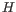) of the paired distances between reads in each set of paired reads (see figure 29.11). Each of these histograms are then used to estimate paired distances as described in the following.

We denote the average number of observations in the histogram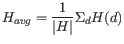where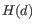is the number of observations (reads) with distance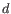and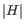is the number of bins in. The gradient ofat distanceis denoted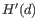. The following algorithm is then used to compute a distance interval for each histogram.

• Identify peaks inas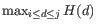where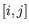is any interval inwhere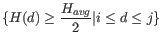.
• For the two largest peaks found, expand the respective intervalsto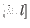where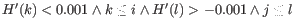. I.e. we search for a point in both directions where the number of observations becomes stable. A window of size 5 is used to calculate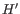in this step.
• Compute the total number of observations in each of the two expanded intervals.
• If only one peak was found, the corresponding intervalis used as the distance estimate unless the peak was at a negative distance in which case no distance estimate is calculated.
• If two peaks were found and the intervalfor the largest peak contains less than 1% of all observations, the distance is not estimated.
• If two peaks were found and the intervalfor the largest peak contain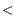2X observations compared to the smaller peak, the distance estimate is only computed if one peak was at a positive distance and the other was at a negative distance. If this is the case the intervalfor the positive peak is used as a distance estimate.
• If two peaks were found and the largest peak has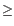2X observations compared to the smaller peak, the intervalcorresponding to the largest peak is used as the distance estimate.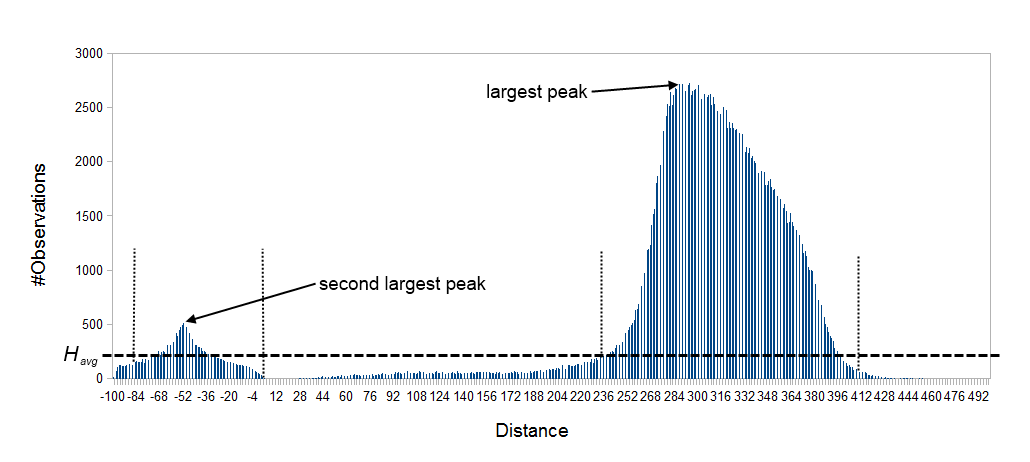Figure 29.11: Histogram of paired distances where tex2html_wrap_inline$H_avg$ is indicated by the horizontal dashed line. There is two peaks, one is at a negative distance while the other larger peak is at a positive distance. The extended interval tex2html_wrap_inline$[k,l]$ for each peak is indicated by the vertical dotted lines.